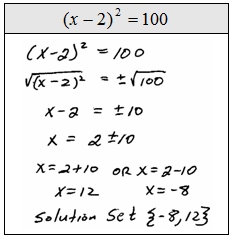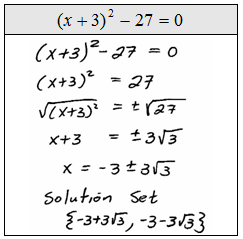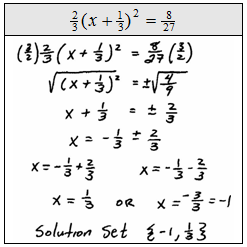## Pages

### Solve by Extracting Square Roots

So far we have been able to solve quadratic equations using the factoring method.The idea was to set the equation to zero, factor it, then set each factor to zero and solve. The zero factor property allowed us to solve factorable quadratic equations.

Solve by factoring.At this point we will learn a new technique that works for quadratic equations that are in the following form: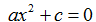The idea here is to isolate the x^2 term and then apply the square root property. For example, ifthen it is fairly clear that x = -3 or x = 3 which can be gotten using the following property: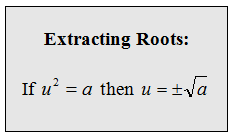When applying the square root to both sides of an equation remember to include the + or -.

Solve by extracting roots:Step 1: Isolate the square term.Step 2: Apply the square root property.Step 3: Be sure to include the + or - and solve as usual.Compare the next three problems to the first three of this section, they are the same questions. Notice that the two very different techniques give us the same solutions.

Solve by extracting roots.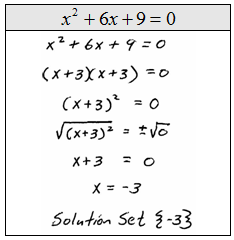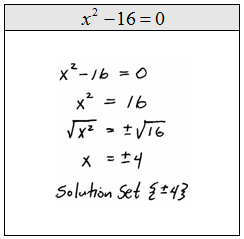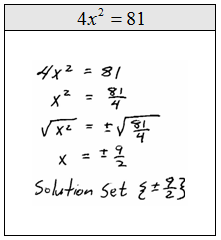Solve.